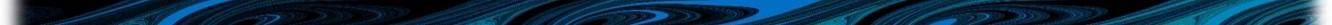# Appendix A Syntax of command descriptions

The manual’s introduction should be reviewed for instructions regarding the use of interactive components deployed in this section.

All WQ Module commands and descriptions are provided in Table B.1. This Appendix describes the syntax of that table.

## A.1 Commands

WQ Module commands are coloured, for example frp adsorption parameters ==. These can be copied and pasted directly into a *.fvwq (or *.fvc where appropriate) control file, and arguments added.

## A.2 Arguments

Descriptions of the arguments expected by WQ Module commands (i.e. information provided after the ==) are contrastingly coloured and always included in angle parentheses, as $$\langle$$argument$$\rangle$$ . This argument content takes one of the following forms:

• A description. For example, for the material == WQ Module command, the argument is described as $$\langle$$Up to 10 comma separated material numbers$$\rangle$$. An example of implementing this description would therefore be

material == 1, 6, 3

• Single keyword options. Options are separated by semicolons. Options need to be used exactly as written because they are keywords. For example, $$\langle$$DO;inorganics;organics$$\rangle$$ describes the three available simulation options for the command simulation class ==. An example of implementing this description would therefore be

simulation class == organics

• Single numeric values. Values are presented using their associated symbol, using the notation described in the manual’s introductory sections. For example, $$F_{sed}^{NO_3}$$ describes the single numeric value for the command nitrate flux ==. An example of implementing this description would therefore be

nitrate flux == 5.7

• Multiple numeric values. Values are presented using their associated symbol, using the notation described in the manual’s introductory sections, and are separated by commas. The number of comma separated symbols indicates the number of expected numeric values. For example, $$\langle K_{sed-O_2}^{NH_4},\theta_{sed}^{NH_4} \rangle$$ describes the two numeric values expected for the command ammonium benthic ==. An example of implementing this description would therefore be

ammonium benthic == 4.2, 1.08

• Combined keyword and numeric value/s options. Arguments are separated by commas. Numeric arguments are represented by their associated symbol, using the notation described in the manual’s introductory sections. Options need to be used exactly as written because they are keywords. Where different keyword options (for the same command) require different numbers or types of corresponding numerical arguments, these are listed as separate table entries for clarity. These different implementations of the same command are however easily navigated between by using the hyperlinked descriptive words in the right hand most table column.

## A.3 Defaults

All WQ Module commands are optional (see Section 4.5), and where not specified by the user, the WQ Module will use library default arguments. These defaults are listed in a separate column of Table B.1. Where defaults are not appropriate, then “N/A” is listed in this column. Initial conditions must be specified via the *.fvc control file and do not have defaults.